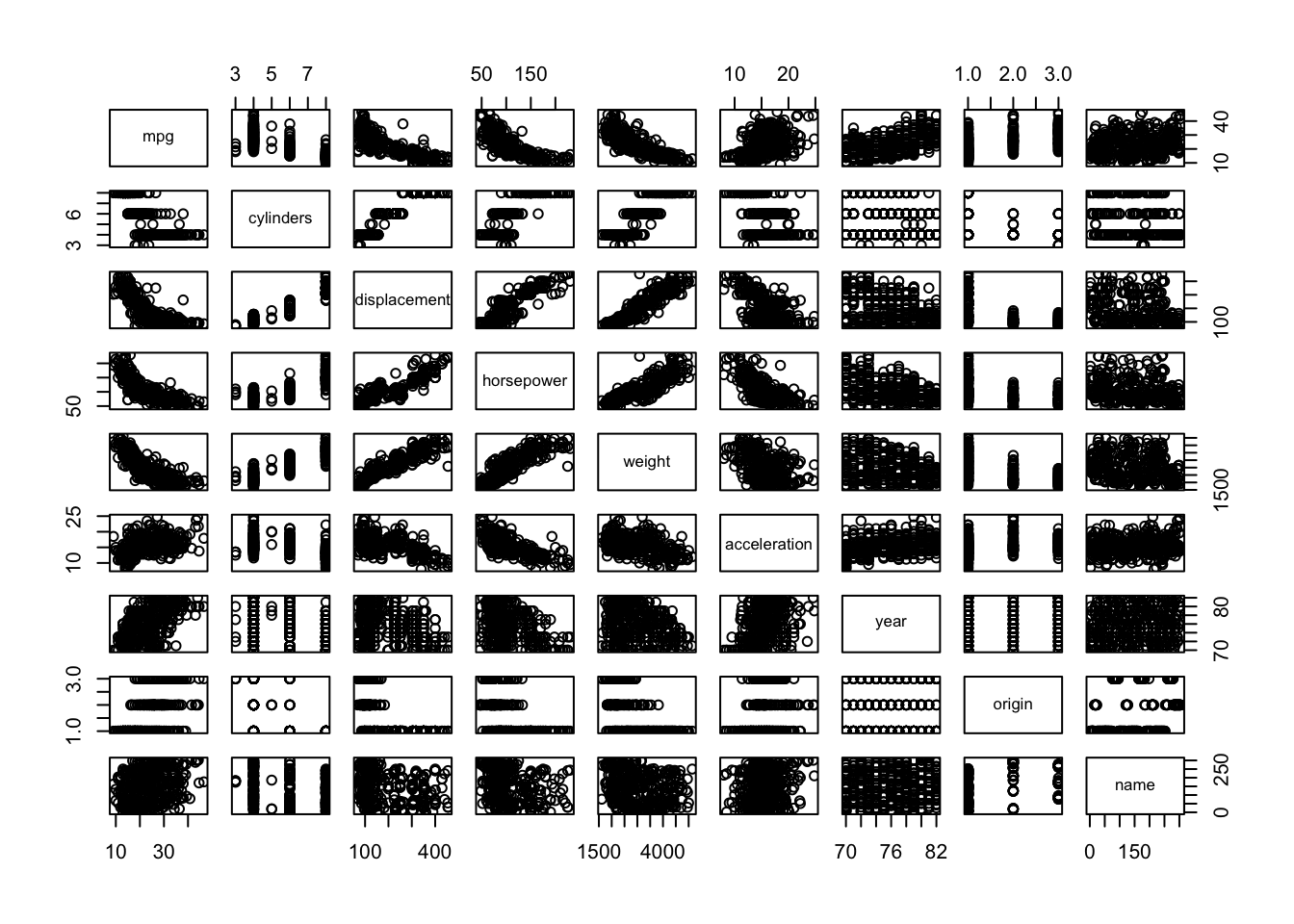## Exercise 4.1

This question involves the use of multiple linear regression on the `Auto` data set. So load the data set from the `ISLR` package first.

If the following code chunk returns an error, you most likely have to install the `ISLR` package first. Use `install.packages("ISLR")` if this is the case.

``data("Auto", package = "ISLR")``
1. Produce a scatterplot matrix which includes all of the variables in the data set.
``pairs(Auto)``1. Compute the matrix of correlations between the variables using the function `cor()`. You will need to exclude the `name` variable, which is qualitative.
``cor(subset(Auto, select = -name))``
``````##                     mpg  cylinders displacement horsepower     weight
## mpg           1.0000000 -0.7776175   -0.8051269 -0.7784268 -0.8322442
## cylinders    -0.7776175  1.0000000    0.9508233  0.8429834  0.8975273
## displacement -0.8051269  0.9508233    1.0000000  0.8972570  0.9329944
## horsepower   -0.7784268  0.8429834    0.8972570  1.0000000  0.8645377
## weight       -0.8322442  0.8975273    0.9329944  0.8645377  1.0000000
## acceleration  0.4233285 -0.5046834   -0.5438005 -0.6891955 -0.4168392
## year          0.5805410 -0.3456474   -0.3698552 -0.4163615 -0.3091199
## origin        0.5652088 -0.5689316   -0.6145351 -0.4551715 -0.5850054
##              acceleration       year     origin
## mpg             0.4233285  0.5805410  0.5652088
## cylinders      -0.5046834 -0.3456474 -0.5689316
## displacement   -0.5438005 -0.3698552 -0.6145351
## horsepower     -0.6891955 -0.4163615 -0.4551715
## weight         -0.4168392 -0.3091199 -0.5850054
## acceleration    1.0000000  0.2903161  0.2127458
## year            0.2903161  1.0000000  0.1815277
## origin          0.2127458  0.1815277  1.0000000``````
1. Use the `lm()` function to perform a multiple linear regression with `mpg` as the response and all other variables except `name` as the predictors. Use the `summary()` function to print the results. Comment on the output. For instance:
``````lm.fit1 <-  lm(mpg ~ . - name, data = Auto)
summary(lm.fit1)``````
``````##
## Call:
## lm(formula = mpg ~ . - name, data = Auto)
##
## Residuals:
##     Min      1Q  Median      3Q     Max
## -9.5903 -2.1565 -0.1169  1.8690 13.0604
##
## Coefficients:
##                Estimate Std. Error t value Pr(>|t|)
## (Intercept)  -17.218435   4.644294  -3.707  0.00024 ***
## cylinders     -0.493376   0.323282  -1.526  0.12780
## displacement   0.019896   0.007515   2.647  0.00844 **
## horsepower    -0.016951   0.013787  -1.230  0.21963
## weight        -0.006474   0.000652  -9.929  < 2e-16 ***
## acceleration   0.080576   0.098845   0.815  0.41548
## year           0.750773   0.050973  14.729  < 2e-16 ***
## origin         1.426141   0.278136   5.127 4.67e-07 ***
## ---
## Signif. codes:  0 '***' 0.001 '**' 0.01 '*' 0.05 '.' 0.1 ' ' 1
##
## Residual standard error: 3.328 on 384 degrees of freedom
## Multiple R-squared:  0.8215, Adjusted R-squared:  0.8182
## F-statistic: 252.4 on 7 and 384 DF,  p-value: < 2.2e-16``````
``i. Is there a relationship between the predictors and the response?``

Yes, there is a relatioship between the predictors and the response by testing the null hypothesis of whether all the regression coefficients are zero. The F-statistic is far from 1 (with a small p-value), indicating evidence against the null hypothesis.

``ii. Which predictors appear to have a statistically significant relationship to the response?``

Looking at the p-values associated with each predictor’s t-statistic, we see that displacement, weight, year, and origin have a statistically significant relationship, while cylinders, horsepower, and acceleration do not.

``iii. What does the coefficient for the `year` variable suggest?``

The regression coefficient for year, `0.7507727`, suggests that for every one year, mpg increases by the coefficient. In other words, cars become more fuel efficient every year by almost 1 mpg / year.

1. Use the `plot()` function to produce diagnostic plots of the linear regression fit. Comment on any problems you see with the fit. Do the residual plots suggest any unusually large outliers? Does the leverage plot identify any observations with unusually high leverage?
``````par(mfrow = c(2, 2))
plot(lm.fit1)``````